# 关于 sql 行转列的感悟<转>

+关注继续查看(1)(2)

Select 姓名 From 1 Group By 姓名代码
Sql语句疑似：
Select 姓名,case  when 科目='语文' then 成绩 end From 成绩表 Group By 姓名

8120，级别 16，状态 1，行 1

'成绩表.成绩' 在选择列表中无效，因为该列既不包含在聚合函数中，也不包含在 GROUP BY 子句中。

8120，级别 16，状态 1，行 1

'成绩表.科目' 在选择列表中无效，因为该列既不包含在聚合函数中，也不包含在 GROUP BY 子句中。

min,avg,sum,count，max和min首先干掉，我们不是要最大值，最小值，难道是平均值？难道是个数？难道是求和？？？

Select 姓名,sum(case when 科目='语文' then 成绩 endFrom 表1 Group By 姓名

Select 姓名,sum(case when 科目='语文' then 成绩 endAs '语文' From表1 Group By 姓名

Select 姓名,

sum(case when 科目='语文' then 成绩 endAs '语文' ,

sum(case when 科目='数学' then 成绩 endAs '数学',

sum(case when 科目='英语' then 成绩 endAs '英语'

From  表1 Group By 姓名

可是结果有NULL，那还不好办，你的case
when then end没写完，还有else没写呢！
Select 姓名,

sum(case when 科目='语文' then 成绩 else 0 endAs '语文' ,

sum(case when 科目='数学' then 成绩 else 0 endAs '数学',

sum(case when 科目='英语' then 成绩 else 0 endAs '英语'

From 表1 Group By 姓名

Select 姓名,

sum(case when 科目='语文' then 成绩 else 0 endAs '语文' ,

sum(case when 科目='数学' then 成绩 else 0 endAs '数学',

sum(case when 科目='英语' then 成绩 else 0 endAs '英语'

From  表1 Group By 姓名

As '语文'”，哪些个“科目='语文'”，其实是数据表中的值，如果仅仅要查询“语文，数学，英语”的话，那么easy啊。
Select 课程 From 表1 Group By 科目

sum(case when 科目='语文' then 成绩 else 0 endAs '语文' ,

sum(case when 科目='数学' then 成绩 else 0 endAs '数学',

sum(case when 科目='英语' then 成绩 else 0 endAs '英语'
……………..

Select ',sum(case when 科目='''+trim(科目)+ ''' then 成绩 else 0 end) As '''+科目+'''' From 表1 Group By 课程

,
sum(case when 科目='语文' then 成绩 else 0 endAs '语文' ,
,
sum(case when 科目='数学' then 成绩 else 0 endAs '数学',
,
sum(case when 科目='英语' then 成绩 else 0 endAs '英语'

declare @sql nvarchar(4000)
set @sql='Select 姓名 '
Select @sql=@sql+',sum(case when 科目='''+科目+ ''' then 成绩 else 0 end) As '''+科目+''''
From表1
Group By 科目
Print @sql

Select 姓名 ,sum(case when 科目='数学' then 成绩 else 0 endAs '数学',sum(case when 科目='英语' then 成绩 else 0 endAs '英语',sum(case when 科目='语文' then 成绩 else 0 endAs '语文'

Select 姓名 ,sum(case when 科目='数学' then 成绩 else 0 endAs '数学',sum(case when 科目='英语' then 成绩 else 0 endAs '英语',sum(case when 科目='语文' then 成绩 else 0 endAs '语文' From 表1 Group By 姓名

@sql 这个变量中了，怎么执行？

SqlServer中有个专门将字符串作为sql语句执行的存储过程
---- sp_executesql。

declare @sql nvarchar(4000)
set @sql='Select 姓名 '
Select @sql=@sql+',sum(case when 科目='''+科目+ ''' then 成绩 else 0 end) As '''+科目+''''

From 成绩表 Group By 科目
Print @sql
set @sql=@sql+' From 成绩表 Group By 姓名'
Print @sql
execute sp_executesql @sql

declare @sql nvarchar(4000)
set @sql='Select 姓名 '
Select @sql=@sql+',sum(case when 科目='''+科目+ ''' then 成绩 else 0 end) As '''+科目+''''

From 科目表
Print @sql
set @sql=@sql+' From 表1 Group By 姓名'
Print @sql
execute sp_executesql @sql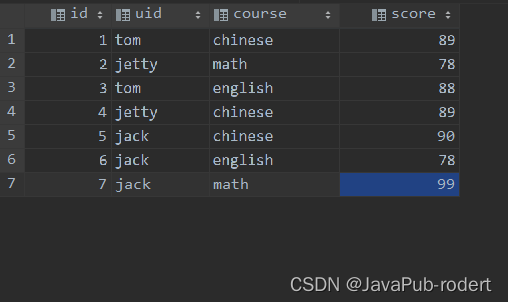63 0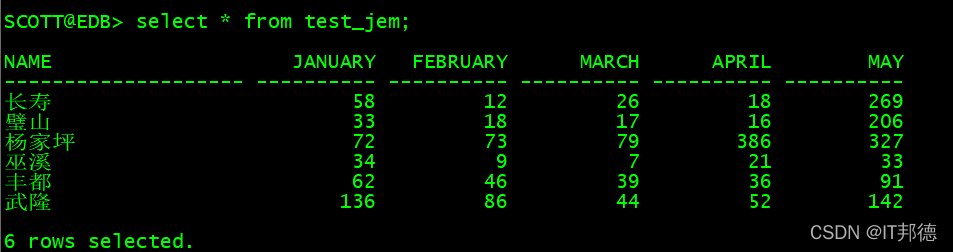84 0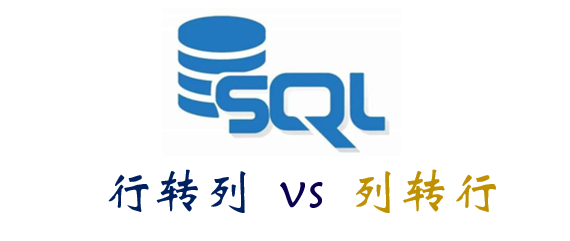SQL中的行转列和列转行
SQL是IT行业很多岗位都要求具备的一项能力，对于数据岗位而言更是如此，甚至说扎实的SQL基础也往往是入职这些岗位的必备技能。而在SQL面试中，一道出镜频率很高的题目就是行转列和列转行的问题，可以说这也是一道经典的SQL题目，本文就这一问题做以介绍分享。
306 0【每日SQL打卡】​​​​​​​​​​​​​​​DAY 19丨行转列【难度中等】​
【每日SQL打卡】​​​​​​​​​​​​​​​DAY 19丨行转列【难度中等】​
17 0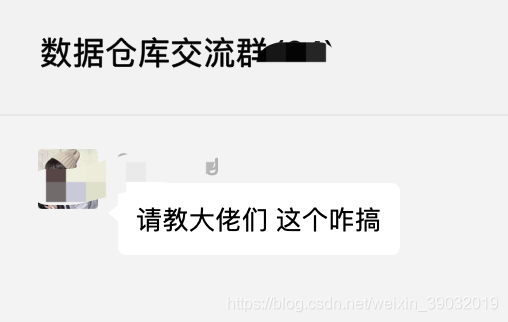178 0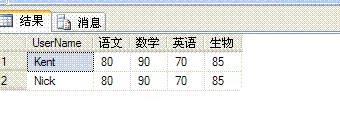1023 0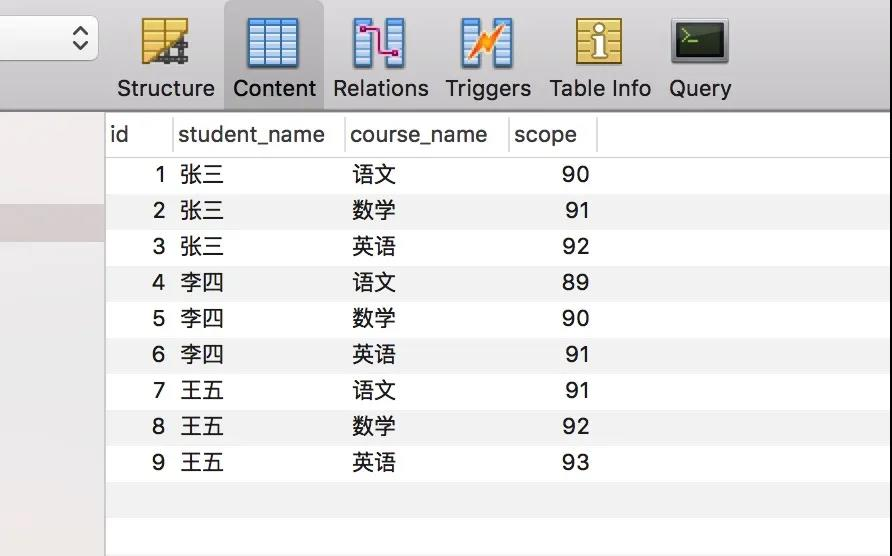67 01907 01097 0470922

SQL进阶及查询219025

SQL入门与实践82366

SQL Server on Linux入门教程427714

SQL完全自学手册348161952

Comparison of Spark SQL with Hive